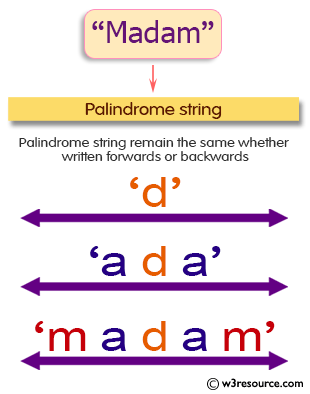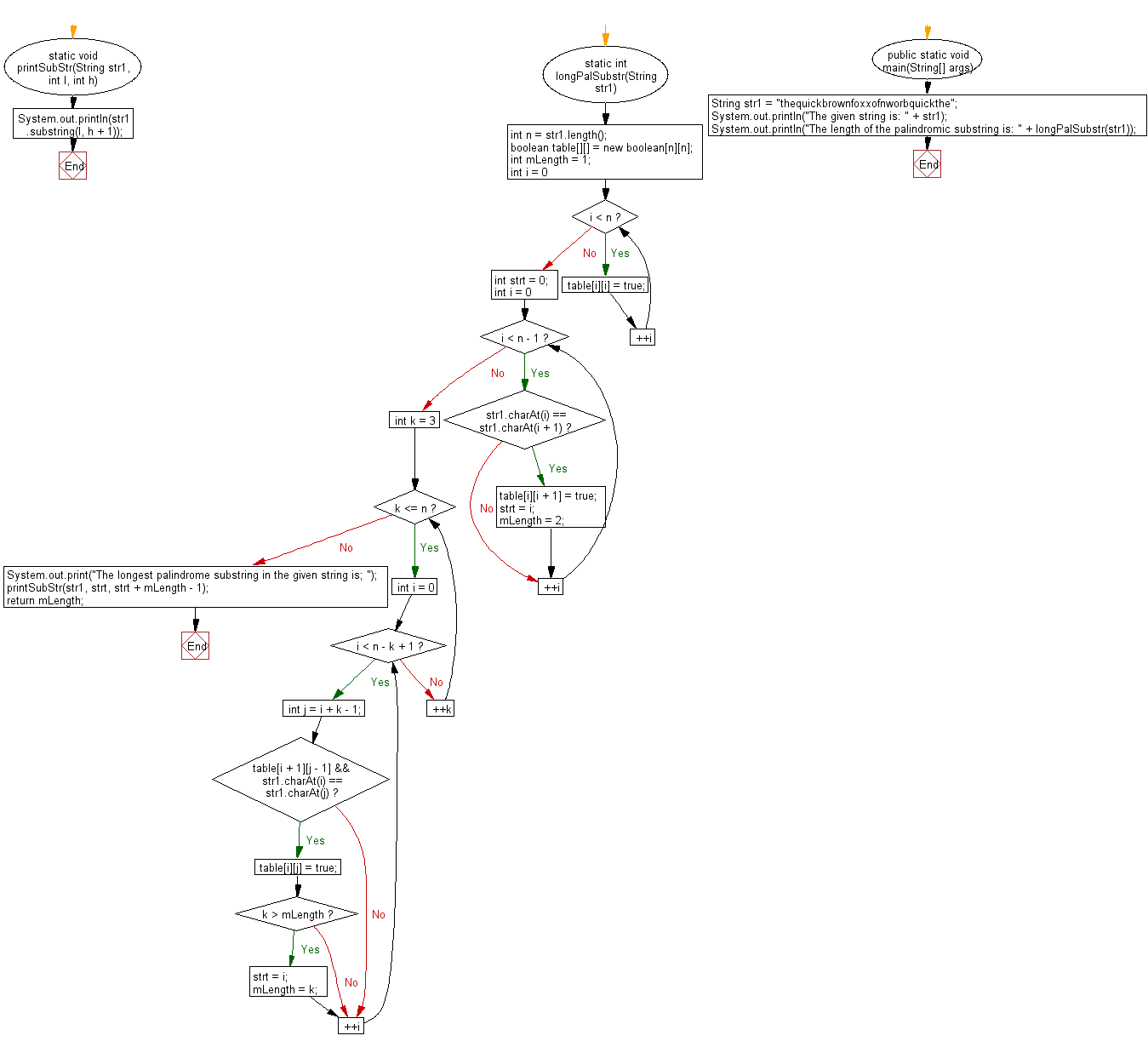﻿ Java exercises: Find longest Palindromic Substring within a string - w3resource# Java String Exercises: Find longest Palindromic Substring within a string

## Java String: Exercise-32 with Solution

Write a Java program to find longest Palindromic Substring within a string.

Pictorial Presentation:Sample Solution:

Java Code:

``````import java.util.*;
public class Main {
static void printSubStr(String str1, int l, int h) {
System.out.println(str1.substring(l, h + 1));
}

static int longPalSubstr(String str1) {
int n = str1.length();
boolean table[][] = new boolean[n][n];
int mLength = 1;
for (int i = 0; i < n; ++i)
table[i][i] = true;
int strt = 0;
for (int i = 0; i < n - 1; ++i) {
if (str1.charAt(i) == str1.charAt(i + 1)) {
table[i][i + 1] = true;
strt = i;
mLength = 2;
}
}
for (int k = 3; k <= n; ++k) {
for (int i = 0; i < n - k + 1; ++i) {
int j = i + k - 1;
if (table[i + 1][j - 1] && str1.charAt(i) == str1.charAt(j)) {
table[i][j] = true;

if (k > mLength) {
strt = i;
mLength = k;
}
}
}
}
System.out.print("The longest palindrome substring in the given string is; ");
printSubStr(str1, strt, strt + mLength - 1);
return mLength;
}
public static void main(String[] args) {
String str1 = "thequickbrownfoxxofnworbquickthe";
System.out.println("The given string is: " + str1);
System.out.println("The length of the palindromic substring is: " + longPalSubstr(str1));
}
}
```
```

Sample Output:

```The given string is: thequickbrownfoxxofnworbquickthe
The longest palindrome substring in the giv
en string is; brownfoxxofnworb
The length of the palindromic substring is: 16
```

Flowchart:Java Code Editor:

Improve this sample solution and post your code through Disqus

What is the difficulty level of this exercise?

﻿

## Java: Tips of the Day

Array vs ArrayLists:

The main difference between these two is that an Array is of fixed size so once you have created an Array you cannot change it but the ArrayList is not of fixed size. You can create instances of ArrayLists without specifying its size. So if you create such instances of an ArrayList without specifying its size Java will create an instance of an ArrayList of default size.

Once an ArrayList is full it re-sizes itself. In fact, an ArrayList is internally supported by an array. So when an ArrayList is resized it will slow down its performance a bit as the contents of the old Array must be copied to a new Array.

At the same time, it's compulsory to specify the size of an Array directly or indirectly while creating it. And also Arrays can store both primitives and objects while ArrayLists only can store objects.

Ref: https://bit.ly/3o8L2KH